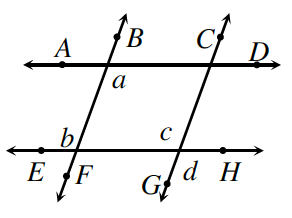### Home > CCG > Chapter Ch7 > Lesson 7.3.1 > Problem7-124

7-124.Carolina compared her proof to Penn’s work in problem 7‑113. Like him, she wanted to prove that if AD EH and BF CG, then a = d. Unfortunately, her statements were in a different order. Examine her proof below and help her decide if her statements are in a logical order in order to prove that $a=d$. Homework Help ✎

 Statements Reasons $\overline{AD}//\overline{EH}$ and $\overline{BF}//\overline{CG}$ Given $a=b$ If lines are parallel, alternate interior angles are equal. $a=c$ Substitution $b=c$ If lines are parallel, corresponding angles are equal. $c=d$ Vertical angles are equal. $a=d$ SubstitutionIs it possible to know that $a=c$ in the 3rd statement? What step is out of order?